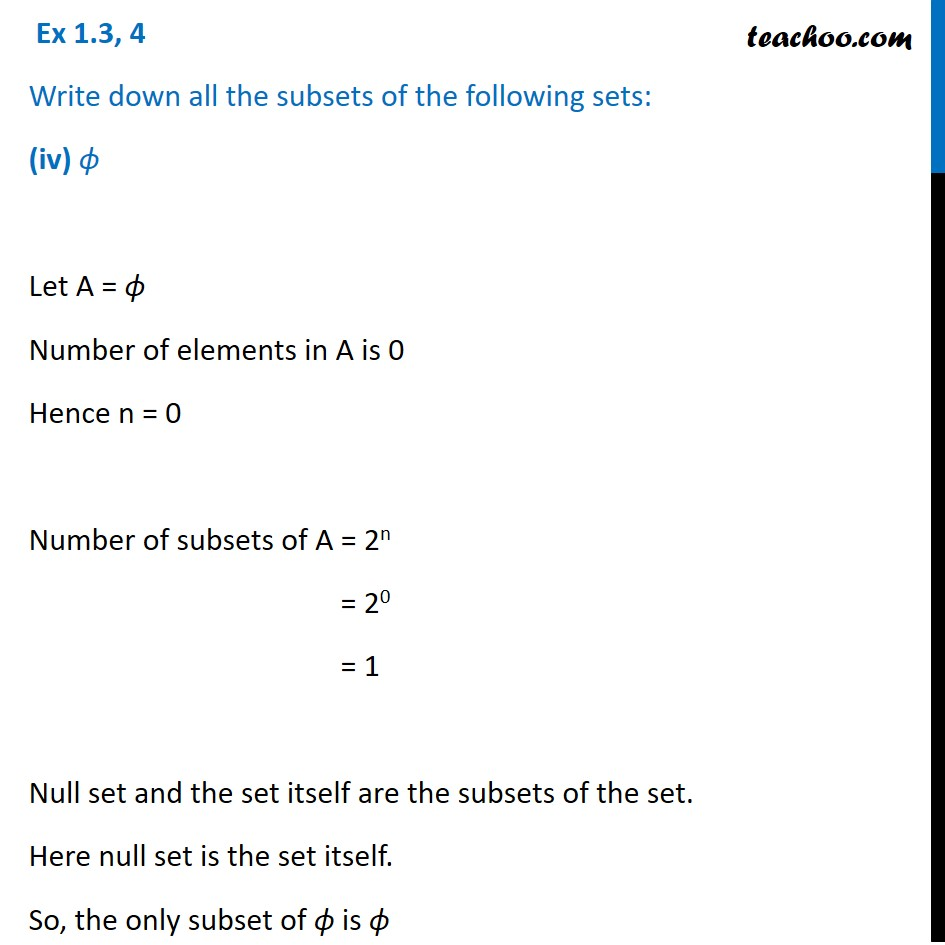1. Chapter 1 Class 11 Sets (Term 1)
2. Serial order wise
3. Ex 1.3

Transcript

Ex 1.3, 4 Write down all the subsets of the following sets: (iv) ϕ Let A = ϕ Number of elements in A is 0 Hence n = 0 Number of subsets of A = 2n = 20 = 1 Null set and the set itself are the subsets of the set. Here null set is the set itself. So, the only subset of ϕ is ϕ